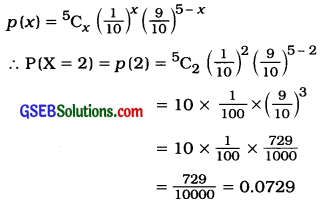# GSEB Solutions Class 12 Statistics Part 2 Chapter 2 Random Variable and Discrete Probability Distribution Probability Ex 2.2

Gujarat Board Statistics Class 12 GSEB Solutions Part 2 Chapter 2 Random Variable and Discrete Probability Distribution Ex 2.2 Textbook Exercise Questions and Answers.

## Gujarat Board Textbook Solutions Class 12 Statistics Part 2 Chapter 2 Random Variable and Discrete Probability Distribution Ex 2.2

Question 1.
For a symmetrical binomial distribution with n = 8, find P(X ≤ 1).
Here, it is given that binomial distribution is symmetric.
∴ P = q = $$\frac{1}{2}$$, n = 8
Putting, n = 8, P = $$\frac{1}{2}$$ and q = $$\frac{1}{2}$$ in
p(x) = nCx px qn – x
p(x) = 8Cx $$\left(\frac{1}{2}\right)^{x}\left(\frac{1}{2}\right)^{8-x}$$
P(X ≤ 1) = P(X = 0, 1)
= [p(0) + p(1)]
= [8C0 $$\left(\frac{1}{2}\right)^{0}\left(\frac{1}{2}\right)^{8}$$ + 8C1 $$\left(\frac{1}{2}\right)^{1}\left(\frac{1}{2}\right)^{7}$$]
= $$\left[\left(\frac{1}{2}\right)^{8}+8\left(\frac{1}{2}\right)^{8}\right]$$
= $$\left[\frac{1}{256}+\frac{8}{256}\right]$$
= $$\frac{9}{256}$$
Hence, P[X ≤ 1] obtained is $$\frac{9}{256}$$.Question 2.
Mean of a binomial distribution is 5 and its variance is equal to the probability of success. Find the parameters of this distribution and hence find the probability of the event of getting none of the failures for this distribution.
Here, mean = np = 5 and variance = npq = p are given.

Putting, np = 5 in
npq = p,
5q = p
Putting, q = 1 – p.
5(1 – p) = p
∴ 5 – 5p = p
∴ 5 = p + 5p
∴ 5 = 6p
∴ p = $$\frac{5}{6}$$

Putting, p = $$\frac{5}{6}$$ in
np = 5
n × $$\frac{5}{6}$$ = 5
∴ n = $$\frac{5 \times 6}{5}$$ = 6
∴ n = 6

So, the parameters of the distribution are
n = 6 and p = $$\frac{5}{6}$$.
q = 1 – p = 1 – $$\frac{5}{6}$$ = $$\frac{1}{6}$$
X = None of the failures. i.e., all successes
∴ x = 6
Putting. n = 6, p = $$\frac{5}{6}$$ and q = $$\frac{1}{6}$$ in
P(x) = nCx pxqn-x
p(x) = 6Cx $$\left(\frac{5}{6}\right)^{x}$$ $$\left(\frac{1}{6}\right)^{6-x}$$
∴ P(X = 6) = p(6) = 6C6 $$\left(\frac{5}{6}\right)^{6}$$ $$\left(\frac{1}{6}\right)^{0}$$
= 1 × $$\frac{15625}{46656}$$ × 1
= $$\frac{15625}{46656}$$
Hence, the probability of getting none of the failures for the given distribution obtained is $$\frac{15625}{46656}$$Question 3.
A person has kept 4 cars to run on rent. The probability that any car is rented during the day is 0.6. Find the probability that more than one but less than 4 cars are rented during a day.
4 cars to run for rent. So n = 4.
X = No. of cars to run for rent is more than one but less than 4.
∴ X = 2 or 3
p = Probability that car is rented during the day = 0.6
∴ q = 1 – p = 1 – 0.6 = 0.4
Putting, n = 4, p = 0.6 and q = 0.4 in
P(X = x) = p(x) = nCx px qn-x
P(X = x) = p(x) = 4Cx (0.6)x (0.4)4 – x
Now, P(X = 2 or X = 3)
= p (2) + p(3)
= 4C2 (0.6)2 (0.4)4-2 + 4C3 (0.6)3 (0.4)4-3
= 6(0.6)2 (0.4)2 + 4(0.6)3 (0.4)1
= 6(0.36) (0.16) + 4(0.216) (0.4)
= 0.3456 + 0.3456
= 0.6912
Hence, the probability that more than one but less than 4 cars rented during a day obtained is 0.6912.

Question 4.
There are 200 farms in a Taluka. Among the bore wells made in these 200 farms of the Taluka, salted water is found in 20 farms. Find the probability of the event of not getting salted water in 3 out of 5 randomly selected farms from the Taluka.
Among the bore wells made in 200 farms, salted water is found in 20 farms.
∴ The probability of getting salted water in the farm is p = $$\frac{20}{200}$$ = $$\frac{1}{10}$$
∴ q = 1 – p = 1 – $$\frac{1}{10}$$ = $$\frac{9}{10}$$
Here, n = 5
X = 3 farms not getting salted water, i. e., 2 farms getting salted water
∴ X = 2
Putting, n = 5, x = 2, p = $$\frac{1}{10}$$ and q = $$\frac{9}{10}$$ in
P(X = x) = p(x) = nCx px qn-xHence, the probability of not getting salted water in 3 farms obtained is 0.0729.Question 5.
An example is given to 6 students to solve. The probability of getting correct solution of the problem by any student is 0.6. Students are trying to solve the problem independently. Find the probability of getting the correct solution by only 2 out of the 6 students.